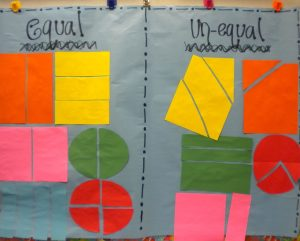# Fractions Part I: Basics KG-2nd grade

by C. Elkins, OK Math and Reading LadyThis is the first post of several I will devote to fractions, starting with basic understanding in first grade and moving up toward operations with fractions in 5th and 6th grade. I would love to hear from you about your students successes and/or difficulties with fractions so I can be sure to address this topic to meet your needs. Free resources below.

What is a fraction?  A fraction represents a part of a whole. It consists of a numerator (which tells how many parts we are describing) and a denominator (how many parts the whole is divided into).

Some basics:

• Fractional parts must be equal. This is a concept introduced in first grade. (See some lesson plan ideas below.)From Pinterest

• The larger the denominator, the smaller the parts AND the smaller the denominator, the larger the parts — when comparing identical sized objects. You can’t compare 1/4 of a cookie with 1/4 of a cake. This is one of the hardest concepts to grasp – so lots of hands-on experience is needed.
• Helpful manipulatives to use with fractions: pattern blocks, color tiles, Cuisenaire rods, fraction circles, fraction strips, fraction bars, graham crackers.
• Be careful about always referring to fractions as “the shaded part.” While this might be true with pictures on worksheets, fractions can be described in these ways also: What fraction of the students are boys? What fraction of the pizza was eaten? What fraction of the candy bar is left? What fraction would belong here on the number line?
• Lines do not necessarily define the fractional part. On the picture shown, the left shows 1/4 shaded. The right also shows 1/4 shaded, but students are likely to say 1/3. Why? Because they count the parts shown (3) and the shaded parts (1) and put that together as a fraction. A way to show this is still 1/4 is to show that the shaded part will fit into the whole shape 4 times.• A unit fraction: This is a fraction with 1 as the numerator (1/4, 1/8, etc.). It is one unit of the whole.
• A fraction that is one unit away from a whole has a numerator one less than the denominator. Examples: 2/3, 3/4, 7/8, 11/12, etc. This is helpful to conceptualize when comparing fractions.
• When reading a fraction number line, compare it to a bar model. Then it is easier to see it is the spaces are the focus, not the tick marks.There are several fraction models students should become familiar with: Area, Set, Bar/Length, and Number line.KG Oklahoma Academic Standard (OAS):

• K.N.3.1:  Distribute equally a set of objects into at least two smaller equal sets.
• “One for you, one for me” – this is essentially the concept of sharing equally with a friend.
• Sort objects into 2 baskets, buckets, tubs, placemats. What happens if there is one left over?
• Make “cookies” with playdough.  Divide between 2 students.
• Discuss this concept when sharing snacks, art supplies, math manipulatives, etc.
• OAS Lesson Plan link for K.N.3.1

First Grade OAS:

• 1.N.3.1 Partition a regular polygon using physical models and recognize when those parts are equal.
• 1.N.3.2 Partition (fair share) sets of objects into equal groupings.
• Sort drawings into 2 groups: Equal vs. Not Equal Free TPT sorting activity
• Investigate different ways to divide a rectangle into equal parts (vertically, horizontally, diagonally).
• Number of lines needed to partition a shape: 1 line divides a shape into 2 parts, 2 lines divide a shape into 3 parts, etc.
• Practice dividing objects into equal sets when sharing manipulatives with partners or small groups (cubes, tiles, base ten, pattern blocks, etc.).
• OAS Lesson Plan link for 1.N.3.1 and 1.N.3.2

Second Grade OAS:

Future posts on fractions will feature these topics: How to accurately partition a shape into fractional parts, equivalent fractions, comparing fractions, literature resources, online resources, using manipulatives, and connections with art. Stay tuned!!

## 2 thoughts on “Fractions Part I: Basics KG-2nd grade”

1.Della Scroggins

I just introduced fractions last week. I will definitely incorporate these ideas as I continue this week with fractions. Thanks.

•Cindy

That’s good timing, isn’t it! Stay tuned for more fraction goodies. I will focus on some third grade fraction concepts next.